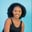Related Tags

python3
numpy

# How to use the np.diff() method for a 2D array in PythonMaria Elijah

## Overview

The numpy.diff() method is used to find the nth order of discrete difference along a specified axis.

Note: In Python, a list of lists can be used to create a two-dimensional (2D) array.

### Syntax

The syntax for the numpy.diff() method is as follows:

numpy.diff(a, n=1, axis=-1)


## Parameters

• a: This represents the input data.

• n: This is an optional parameter. It denotes the number of times the difference value is calculated.

• axis: This is an optional parameter. It denotes the axis over which the difference is calculated.

### Return value

The numpy.diff() method returns the nth order of discrete difference along a given axis.

### Example

The following code shows how to use the numpy.diff() method for two-dimensional (2D) arrays.

# import numpy
import numpy as np
# create list
x1 = [3,4,8]
x2 = [2,6,5]
# convert the lists to 2D array using np.array
a = np.array([x1,x2])

# compute the n-th discrete order difference
# and store the result in result
result = np.diff(a, axis=0)

print(result)
Using the np.floor_divide() function

### Explanation

The following is the explanation for the code above:

• Line 2: We import the numpy library.
• Lines 4 and 5: We create two lists called x1 and x2.
• Line 7: We use the np.array() method to convert the lists to a 2D array.
• Line 11: We use np.diff() to compute the nth discrete difference along axis=0. The result is stored in a new variable called result.
• Line 13: We display the result.

RELATED TAGS

python3
numpy

CONTRIBUTORMaria Elijah
RELATED COURSES

View all Courses

Keep Exploring

Learn in-demand tech skills in half the time